The OpenFOAM FoundationchemPointISAT< CompType, ThermoType > Class Template Reference

Leaf of the binary tree. The chemPoint stores the composition 'phi', the mapping of this composition Rphi, the mapping gradient matrix A and the matrix describing the Ellipsoid Of Accuracy (EOA). More...

## Public Member Functions

chemPointISAT (TDACChemistryModel< CompType, ThermoType > &chemistry, const scalarField &phi, const scalarField &Rphi, const scalarSquareMatrix &A, const scalarField &scaleFactor, const scalar &tolerance, const label &completeSpaceSize, const dictionary &coeffsDict, binaryNode< CompType, ThermoType > *node=nullptr)
Construct from components. More...

chemPointISAT (const chemPointISAT< CompType, ThermoType > &p, binaryNode< CompType, ThermoType > *node)
Construct from another chemPoint and reference to a binary node. More...

chemPointISAT (chemPointISAT< CompType, ThermoType > &p)
Construct from another chemPoint. More...

TDACChemistryModel< CompType, ThermoType > & chemistry ()

label nGrowth ()

labelcompleteSpaceSize ()

const scalarFieldphi () const

const scalarFieldRphi () const

const scalarFieldscaleFactor ()

const scalar & tolerance ()

binaryNode< CompType, ThermoType > *& node ()

const scalarSquareMatrixA () const

scalarSquareMatrixA ()

const scalarSquareMatrixLT () const

scalarSquareMatrixLT ()

label nActiveSpecies ()

List< label > & completeToSimplifiedIndex ()

List< label > & simplifiedToCompleteIndex ()

void increaseNumRetrieve ()
Increases the number of retrieves the chempoint has generated. More...

void resetNumRetrieve ()
Resets the number of retrieves at each time step. More...

Increases the "counter" of the chP life. More...

label simplifiedToCompleteIndex (const label i)

const labeltimeTag ()

labellastTimeUsed ()

bool & toRemove ()

labelmaxNumNewDim ()

const labelnumRetrieve ()

bool variableTimeStep () const

bool inEOA (const scalarField &phiq)
To RETRIEVE the mapping from the stored chemPoint phi, the query. More...

bool grow (const scalarField &phiq)
More details about the minimum-volume ellipsoid covering an. More...

bool checkSolution (const scalarField &phiq, const scalarField &Rphiq)
If phiq is not in the EOA, then the mapping is computed. More...

## Static Public Member Functions

static void changeTolerance (scalar newTol)

## Detailed Description

### template<class CompType, class ThermoType> class Foam::chemPointISAT< CompType, ThermoType >

Leaf of the binary tree. The chemPoint stores the composition 'phi', the mapping of this composition Rphi, the mapping gradient matrix A and the matrix describing the Ellipsoid Of Accuracy (EOA).

1) When the chemPoint is created the region of accuracy is approximated by an ellipsoid E centered in 'phi' (obtained with the constant): E = {x| ||L^T.(x-phi)|| <= 1}, with x a point in the composition space and L^T the transpose of an upper triangular matrix describing the EOA (see below: "Computation of L" ).

2) To RETRIEVE the mapping from the chemPoint phi, the query point phiq has to be in the EOA of phi. It follows that, dphi=phiq-phi and to test if phiq is in the ellipsoid there are two methods. First, compare r=||dphi|| with rmin and rmax. If r < rmin, phiq is in the EOA. If r > rmax, phiq is out of the EOA. This operations is O(completeSpaceSize) and is performed first. If rmin < r < rmax, then the second method is used: ||L^T.dphi|| <= 1 to be in the EOA. If phiq is in the EOA, Rphiq is obtained by linear interpolation: Rphiq= Rphi + A.dphi.

3) If phiq is not in the EOA, then the mapping is computed. But as the EOA is a conservative approximation of the region of accuracy surrounding the point phi, we could expand it by comparing the computed results with the one obtained by linear interpolation. The error epsGrow is calculated: epsGrow = ||B.(dR - dRl)||, with dR = Rphiq - Rphi, dRl = A.dphi and B the diagonal scale factor matrix. If epsGrow <= tolerance, the EOA is too conservative and a GROW is performed otherwise, the newly computed mapping is associated to the initial composition and added to the tree.

4) To GROW the EOA, we expand it to include the previous EOA and the query point phiq. The rank-one matrix method is used. The EOA is transformed to a hypersphere centered at the origin. Then it is expanded to include the transformed point phiq' on its boundary. Then the inverse transformation give the modified matrix L' (see below: "Grow the EOA").

Computation of L : In , the EOA of the constant approximation is given by E = {x| ||B.A/tolerance.(x-phi)|| <= 1},

with B a scale factor diagonal matrix, A the mapping gradient matrix and tolerance the absolute tolerance. If we take the QR decomposition of (B.A)/tolerance= Q.R, with Q an orthogonal matrix and R an upper triangular matrix such that the EOA is described by (phiq-phi0)^T.R^T.R.(phiq-phi0) <= 1 L^T = R, both Cholesky decomposition of A^T.B^T.B.A/tolerance^2 This representation of the ellipsoid is used in  and in order to avoid large value of semi-axe length in certain direction, a Singular Value Decomposition (SVD) is performed on the L matrix: L = UDV^T, with the orthogonal matrix U giving the directions of the principal axes and 1/di the inverse of the element of the diagonal matrix D giving the length of the principal semi-axes. To avoid very large value of those length, di' = max(di, 1/(alphaEOA*sqrt(tolerance))), with alphaEOA = 0.1 (see ) di' = max(di, 1/2), see . The latter will be used in this implementation. And L' = UD'V^T, with D' the diagonal matrix with the modified di'.

Grow the EOA : More details about the minimum-volume ellipsoid covering an ellipsoid E and a point p are found in . Here is the main steps to obtain the modified matrix L' describing the new ellipsoid.

1) calculate the point p' in the transformed space : p' = L^T.(p-phi) 2) compute the rank-one decomposition: G = I + gamma.p'.p'^T, with gamma = (1/|p'|-1)*1/|p'|^2 3) compute L': L' = L.G.

References:

```     Pope, S. B. (1997).
Computationally efficient implementation of combustion chemistry using
Combustion Theory and Modelling, 1, 41-63.

 Lu, L., & Pope, S. B. (2009).
An improved algorithm for in situ adaptive tabulation.
Journal of Computational Physics, 228(2), 361-386.

 Pope, S. B. (2008).
Algorithms for ellipsoids.
Cornell University Report No. FDA, 08-01.
```

Definition at line 143 of file chemPointISAT.H.

## ◆ chemPointISAT() [1/3]

 chemPointISAT ( TDACChemistryModel< CompType, ThermoType > & chemistry, const scalarField & phi, const scalarField & Rphi, const scalarSquareMatrix & A, const scalarField & scaleFactor, const scalar & tolerance, const label & completeSpaceSize, const dictionary & coeffsDict, binaryNode< CompType, ThermoType > * node = `nullptr` )

Construct from components.

Definition at line 201 of file chemPointISAT.C.

Here is the call graph for this function: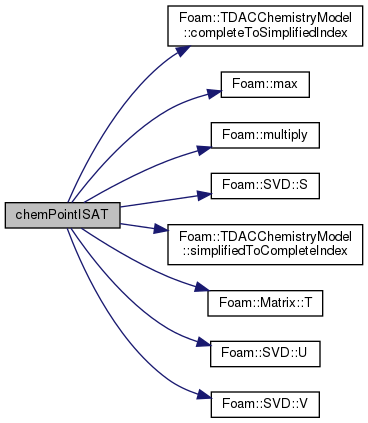## ◆ chemPointISAT() [2/3]

 chemPointISAT ( const chemPointISAT< CompType, ThermoType > & p, binaryNode< CompType, ThermoType > * node )

Construct from another chemPoint and reference to a binary node.

## ◆ chemPointISAT() [3/3]

 chemPointISAT ( Foam::chemPointISAT< CompType, ThermoType > & p )

Construct from another chemPoint.

Definition at line 320 of file chemPointISAT.C.

Here is the call graph for this function: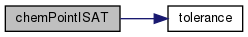## ◆ chemistry()

 TDACChemistryModel& chemistry ( )
inline

Definition at line 278 of file chemPointISAT.H.

Referenced by binaryNode< CompType, ThermoType >::calcV().

Here is the caller graph for this function: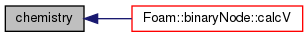## ◆ nGrowth()

 label nGrowth ( )
inline

Definition at line 283 of file chemPointISAT.H.

Referenced by ISAT< CompType, ThermoType >::~ISAT().

Here is the caller graph for this function: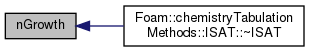## ◆ completeSpaceSize()

 label& completeSpaceSize ( )
inline

Definition at line 288 of file chemPointISAT.H.

Referenced by binaryNode< CompType, ThermoType >::calcV().

Here is the caller graph for this function: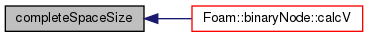## ◆ phi()

 const scalarField& phi ( ) const
inline

Definition at line 293 of file chemPointISAT.H.

Here is the caller graph for this function: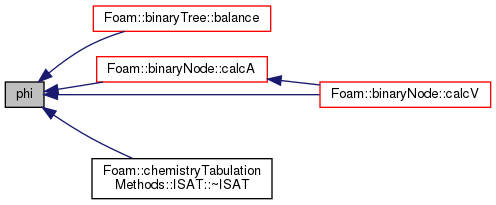## ◆ Rphi()

 const scalarField& Rphi ( ) const
inline

Definition at line 298 of file chemPointISAT.H.

Referenced by ISAT< CompType, ThermoType >::~ISAT().

Here is the caller graph for this function: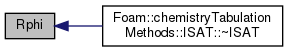## ◆ scaleFactor()

 const scalarField& scaleFactor ( )
inline

Definition at line 303 of file chemPointISAT.H.

Referenced by binaryNode< CompType, ThermoType >::calcV().

Here is the caller graph for this function: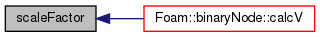## ◆ tolerance()

 const scalar& tolerance ( )
inline

Definition at line 308 of file chemPointISAT.H.

Here is the caller graph for this function: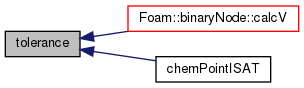## ◆ changeTolerance()

 static void changeTolerance ( scalar newTol )
inlinestatic

Definition at line 313 of file chemPointISAT.H.

## ◆ node()

 binaryNode*& node ( )
inline

Definition at line 318 of file chemPointISAT.H.

Here is the caller graph for this function: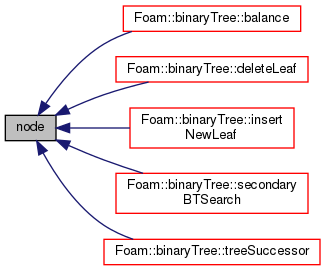## ◆ A() [1/2]

 const scalarSquareMatrix& A ( ) const
inline

Definition at line 323 of file chemPointISAT.H.

Referenced by ISAT< CompType, ThermoType >::~ISAT().

Here is the caller graph for this function: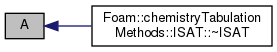## ◆ A() [2/2]

 scalarSquareMatrix& A ( )
inline

Definition at line 328 of file chemPointISAT.H.

## ◆ LT() [1/2]

 const scalarSquareMatrix& LT ( ) const
inline

Definition at line 333 of file chemPointISAT.H.

Referenced by binaryNode< CompType, ThermoType >::calcV().

Here is the caller graph for this function: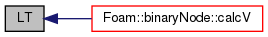## ◆ LT() [2/2]

 scalarSquareMatrix& LT ( )
inline

Definition at line 338 of file chemPointISAT.H.

## ◆ nActiveSpecies()

 label nActiveSpecies ( )
inline

Definition at line 343 of file chemPointISAT.H.

Here is the caller graph for this function: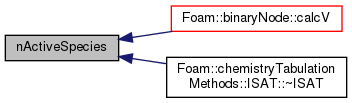## ◆ completeToSimplifiedIndex()

 List
inline

Definition at line 348 of file chemPointISAT.H.

Here is the caller graph for this function: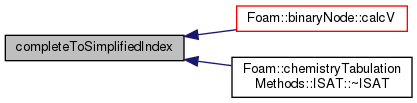## ◆ simplifiedToCompleteIndex() [1/2]

 List
inline

Definition at line 353 of file chemPointISAT.H.

Here is the call graph for this function:## ◆ increaseNumRetrieve()

 void increaseNumRetrieve ( )

Increases the number of retrieves the chempoint has generated.

Definition at line 789 of file chemPointISAT.C.

Here is the caller graph for this function: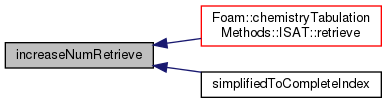## ◆ resetNumRetrieve()

 void resetNumRetrieve ( )

Resets the number of retrieves at each time step.

Definition at line 796 of file chemPointISAT.C.

Here is the caller graph for this function: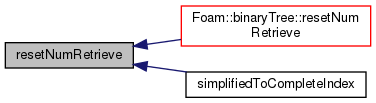Increases the "counter" of the chP life.

Definition at line 803 of file chemPointISAT.C.

Here is the caller graph for this function: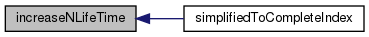## ◆ simplifiedToCompleteIndex() [2/2]

 Foam::label simplifiedToCompleteIndex ( const label i )

Definition at line 812 of file chemPointISAT.C.

## ◆ timeTag()

 const label& timeTag ( )
inline

Definition at line 369 of file chemPointISAT.H.

Here is the caller graph for this function:## ◆ lastTimeUsed()

 label& lastTimeUsed ( )
inline

Definition at line 374 of file chemPointISAT.H.

## ◆ toRemove()

 bool& toRemove ( )
inline

Definition at line 379 of file chemPointISAT.H.

Here is the caller graph for this function:## ◆ maxNumNewDim()

 label& maxNumNewDim ( )
inline

Definition at line 384 of file chemPointISAT.H.

## ◆ numRetrieve()

 const label& numRetrieve ( )
inline

Definition at line 389 of file chemPointISAT.H.

inline

Definition at line 394 of file chemPointISAT.H.

## ◆ variableTimeStep()

 bool variableTimeStep ( ) const
inline

Definition at line 399 of file chemPointISAT.H.

Referenced by binaryNode< CompType, ThermoType >::binaryNode().

Here is the call graph for this function: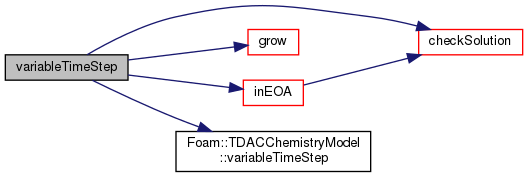Here is the caller graph for this function: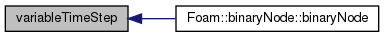## ◆ inEOA()

 bool inEOA ( const scalarField & phiq )

To RETRIEVE the mapping from the stored chemPoint phi, the query.

point phiq has to be in the EOA of phi. To test if phiq is in the ellipsoid: ||L^T.dphi|| <= 1

Definition at line 365 of file chemPointISAT.C.

Here is the call graph for this function: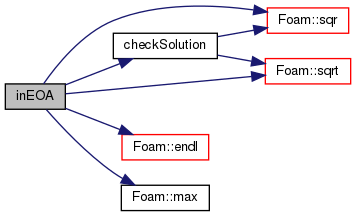Here is the caller graph for this function: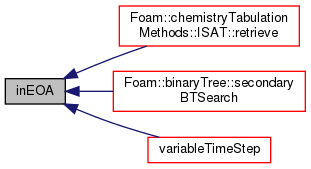## ◆ grow()

 bool grow ( const scalarField & phiq )

More details about the minimum-volume ellipsoid covering an.

ellipsoid E and a point p are found in . Here is the main steps to obtain the modified matrix L' describing the new ellipsoid. 1) calculate the point p' in the transformed space : p' = L^T.(p-phi) 2) compute the rank-one decomposition: G = I + gamma.p'.p'^T, with gamma = (1/|p'|-1)*1/|p'|^2 3) compute L': L'L'^T = (L.G)(L.G)^T, L'^T is then obtained by QR decomposition of (L.G)^T = G^T.L^T  Stephen B. Pope, "Algorithms for ellipsoids", FDA 08-01, Cornell University, 2008

add new column and line for the new active species transfer last two lines of the previous matrix (p and T) to the end

(change the diagonal position) !set all element of the new lines and columns to zero except diagonal /*! (=1/(tolerance*scaleFactor))

Definition at line 604 of file chemPointISAT.C.

Here is the call graph for this function: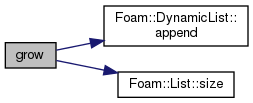Here is the caller graph for this function: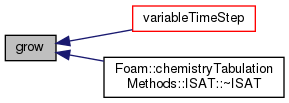## ◆ checkSolution()

 bool checkSolution ( const scalarField & phiq, const scalarField & Rphiq )

If phiq is not in the EOA, then the mapping is computed.

But as the EOA is a conservative approximation of the region of accuracy surrounding the point phi, we could expand it by comparing the computed results with the one obtained by linear interpolation. The error eps is calculated: eps = ||B.(dR - dRl)||, with dR = Rphiq - Rphi, dRl = A.dphi and B the diagonal scale factor matrix. If eps <= tolerance, the EOA is too conservative and a GROW is performed, otherwise, the newly computed mapping is associated to the initial composition and added to the tree.

Definition at line 533 of file chemPointISAT.C.

References phi, Foam::sqr(), and Foam::sqrt().

Here is the call graph for this function:Here is the caller graph for this function: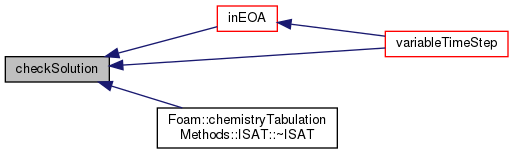The documentation for this class was generated from the following files:
• src/thermophysicalModels/chemistryModel/chemistryModel/TDACChemistryModel/tabulation/ISAT/chemPointISAT/chemPointISAT.H
• src/thermophysicalModels/chemistryModel/chemistryModel/TDACChemistryModel/tabulation/ISAT/chemPointISAT/chemPointISAT.C